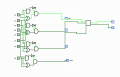# Designing a logic circuit using a full adder?

#### ronBrown

Joined May 1, 2020
4
how would i go about designing a logic circuit to find the number of 1's in a 4 bit number with the output being a 3 bit number using a full adder?

#### WBahn

Joined Mar 31, 2012
26,398
Greetings and welcome to AAC. Since this is Homework Help and not Homework Done For You, you need to show your best attempt to solve your homework assignment.

Try a slightly simpler problem first involving a 3-bit number and a 2-bit result.

#### ronBrown

Joined May 1, 2020
4
Greetings and welcome to AAC. Since this is Homework Help and not Homework Done For You, you need to show your best attempt to solve your homework assignment.

Try a slightly simpler problem first involving a 3-bit number and a 2-bit result.
thank you, i believe i have that part working but my issue is how exactly should i go about just adding the last 1 bit number. would an half adder be appropriate here? here is my circuit btw#### WBahn

Joined Mar 31, 2012
26,398
What's the square box over on the right? A full adder? Would be nice if there was some indication of that, if it is.

What's this x1 and y3 nonsense?

You have a single four-bit input, not two.

What would you do if asked to simply add two 1-bit numbers to produce a 2-bit result?

What would you do if asked to simply add two 2-bit numbers to produce a 3-bit result?

Could you use the circuits that do these two things to add four 1-bit numbers to produce a 3-bit result?

#### ronBrown

Joined May 1, 2020
4
yes the square box is a full adder. the purpose of the circuit is to XOR 2 4-bit numbers or 8 bits. That is what the values of x0 and y0 represent. The corresponding values being xor to then get the final output of bits that would be the hamming distance if we just count the number of ones.

#### WBahn

Joined Mar 31, 2012
26,398
yes the square box is a full adder. the purpose of the circuit is to XOR 2 4-bit numbers or 8 bits. That is what the values of x0 and y0 represent. The corresponding values being xor to then get the final output of bits that would be the hamming distance if we just count the number of ones.
Why are you throwing needless complexity at this? There is nothing in the problem statement, as you provided it, that says anything about Hamming distances.

Be that as it may, can you see that the output of your bank of XOR gates is nothing more than four 1-bit numbers that you want to sum up?

What would you do if asked to simply add two 1-bit numbers to produce a 2-bit result?

What would you do if asked to simply add two 2-bit numbers to produce a 3-bit result?